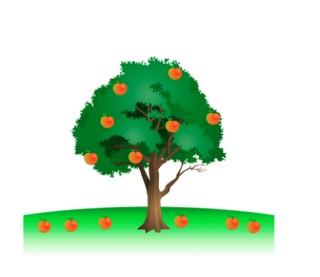Subtraction with numbers up to 20

# Subtraction with numbers up to 20

Students subtract with numbers up to 208,000 schools use Gynzy92,000 teachers use Gynzy1,600,000 students use Gynzy

## General

Students learn to subtract with numbers up to 20. They practice in visual, abstract and story form.

## Standards

CCSS.Math.Content.1.OA.C

## Learning objective

Students will be able to subtract with numbers up to 20.

## Introduction

The interactive whiteboard shows a number line showing a number of jumps which ask students to add up. Ask students which numbers should be written in the boxes below the number line. Then show an ice cube tray and ask students to count the ice cubes. Ask students if there is an easy way to count this number. Place the answer in the tens-ones chart.

## Instruction

This lesson covers subtraction in three forms, in the visual, abstract and story form. You may choose to skip to the part that is best suited to your classroom needs or go through them one by one. Starting with the visual, discuss the steps of subtraction with your students. First you count the total number of objects, this is the first number in your subtraction problem (the minuend). Then you count how many are taken away, this is the second number of your subtraction problem (the subtrahend). When you take the second away from the first you are left with the difference. Demonstrate this with the ice cubes and the candles. Check that students are able to do this by asking them to subtract the cans and bananas. Then move to the abstract and discuss the different strategies they can use for subtraction. You could use a rekenrek or blocks, you can count back on a number line, or you could also see how many you need to add to the second number (subtrahend) to equal the first number (minuend). You can also subtract to an easier number (like ten) and subtract in two steps. Have students find the difference of the next subtraction problems. Ask them to explain their strategy. Finally walk students through the steps of solving a story problem. Make sure students know how to determine which numbers are needed for the math problem, what kind of math problem it is, to say or write the math problem, and finally to solve the math problem. Practice this as a class and then ask students to solve a story problem.

Check that students are able to subtract with numbers up to 20 by asking the following questions:
- What do you look at when you solve a story problem?
- How do you solve 13 - 4?

## Quiz

Students are given ten problems in which to practice subtraction to 20. They are given visual, abstract, and story problems to solve.

## Closing

Discuss with students that it is important to be able to subtract with numbers up to 20 so they are able to calculate and determine how many objects they have left. On the interactive whiteboard, show the students an image and ask them to determine what the subtraction problem is, and then to solve it individually or in pairs. Then, show the subtraction problems on the board and ask students to drag the problems to their differences.

## Teaching tips

Students who have difficulty with this learning goal can be supported by the use of a number line or manipulatives like MAB blocks to follow the steps of subtraction. Determining how many there are, and how many are taken away, and then finding the difference.

## Instruction materials

Math manipulatives like MAB blocks.

### The online teaching platform for interactive whiteboards and displays in schools

• Save time building lessons

• Manage the classroom more efficiently

• Increase student engagement